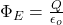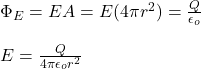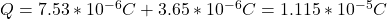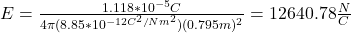## A thin, metallic spherical shell of radius 0.347 m0.347 m has a total charge of 7.53×10−6 C7.53×10−6 C placed on it. A point charge of 3.65×

Question

A thin, metallic spherical shell of radius 0.347 m0.347 m has a total charge of 7.53×10−6 C7.53×10−6 C placed on it. A point charge of 3.65×10−6 C3.65×10−6 C is placed at the center of the shell. What is the electric field magnitude EE a distance 0.795 m0.795 m from the center of the spherical shell?

in progress 0
2 weeks 2021-08-29T02:55:03+00:00 1 Answers 0 views 0

E = 12640.78 N/C

Explanation:

In order to calculate the electric field you can use the Gaussian theorem.

Thus, you have:ФE: electric flux trough the Gaussian surface

Q: net charge inside the Gaussian surface

εo: dielectric permittivity of vacuum = 8.85*10^-12 C^2/Nm^2

If you take the Gaussian surface as a spherical surface, with radius r, the electric field is parallel to the surface anywhere. Then, you have:r can be taken as the distance in which you want to calculate the electric field, that is, 0.795m

Next, you replace the values of the parameters in the last expression, by taking into account that the net charge inside the Gaussian surface is:Finally, you obtain for E:hence, the electric field at 0.795m from the center of the spherical shell is 12640.78 N/C